• Slides: 20Comunicaciones Digitales Avanzadas EL 7041 Comunicaciones Digitales Avanzadas César Azurdia Meza, Ph. D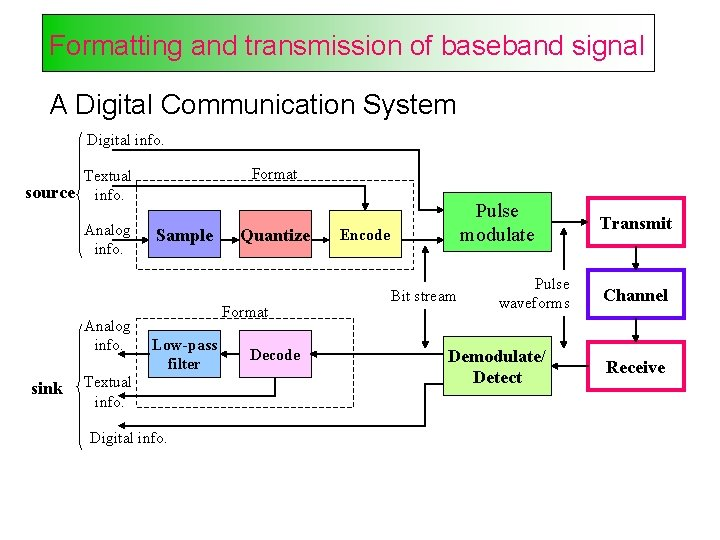Formatting and transmission of baseband signal A Digital Communication System Digital info. Format Textual source info. Analog info. sink Sample Quantize Format Low-pass filter Textual info. Digital info. Decode Pulse modulate Encode Bit stream Pulse waveforms Demodulate/ Detect Transmit Channel ReceiveFormat analog signals To transform an analog waveform into a form that is compatible with a digital communication system, the following steps are taken: 1. Sampling 2. Quantization and encoding 3. Baseband transmissionSampling Time domain Frequency domain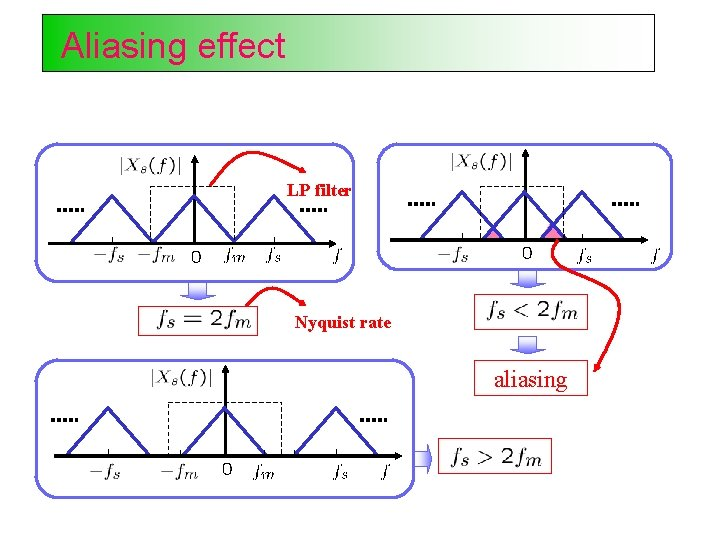Aliasing effect LP filter Nyquist rate aliasingSampling theorem Analog signal Sampling process Pulse amplitude modulated (PAM) signal Sampling theorem: A band-limited signal with no spectral components beyond , can be uniquely determined by values sampled at uniform intervals of The sampling rate, called the Nyquist rate. is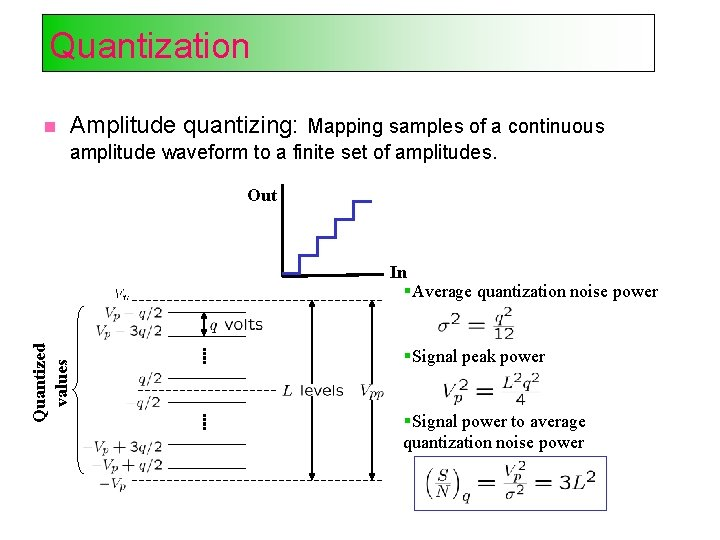Quantization Amplitude quantizing: Mapping samples of a continuous amplitude waveform to a finite set of amplitudes. Out Quantized values In Average quantization noise power Signal peak power Signal power to average quantization noise powerEncoding (PCM) A uniform linear quantizer is called Pulse Code Modulation (PCM). Pulse code modulation (PCM): Encoding the quantized signals into a digital word (PCM word or codeword). Each quantized sample is digitally encoded into an l bits codeword where L in the number of quantization levels and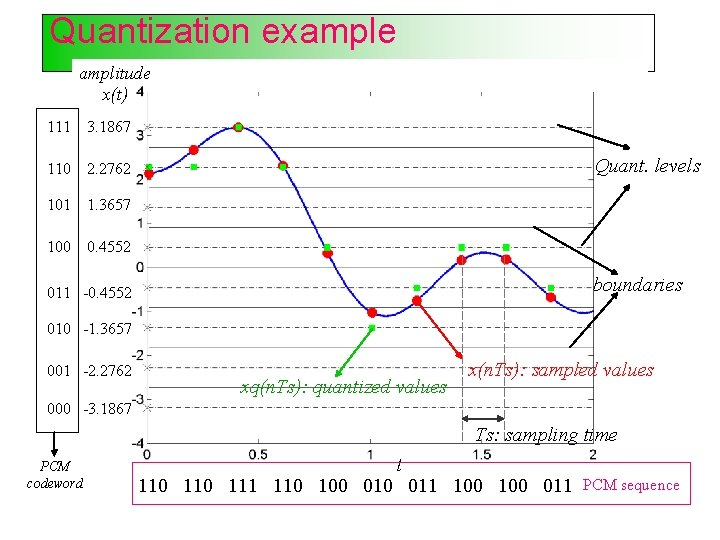Quantization example amplitude x(t) 111 3. 1867 110 2. 2762 101 1. 3657 100 0. 4552 Quant. levels boundaries 011 -0. 4552 010 -1. 3657 001 -2. 2762 xq(n. Ts): quantized values x(n. Ts): sampled values 000 -3. 1867 Ts: sampling time PCM codeword t 110 111 110 100 011 PCM sequence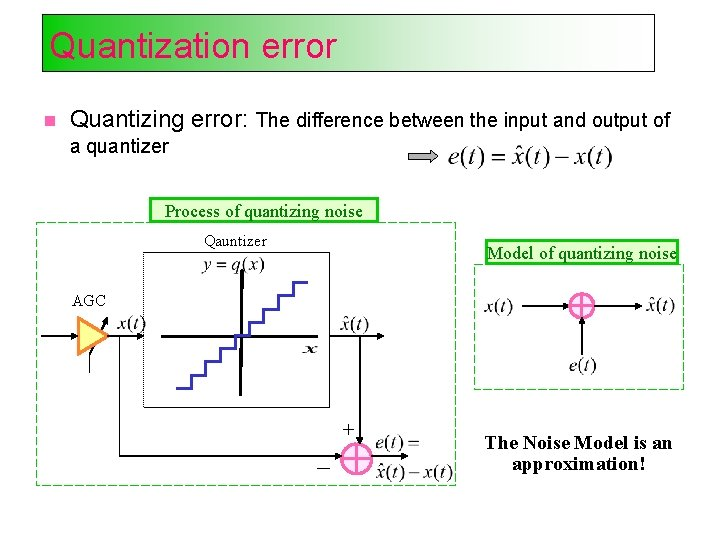Quantization error Quantizing error: The difference between the input and output of a quantizer Process of quantizing noise Qauntizer Model of quantizing noise AGC + The Noise Model is an approximation!Quantization error … Quantizing error: Granular or linear errors happen for inputs within the dynamic range of quantizer Saturation errors happen for inputs outside the dynamic range of quantizer Saturation errors are larger than linear errors (AKA as “Overflow” or “Clipping”) Saturation errors can be avoided by proper tuning of AGC Saturation errors need to be handled by Overflow Detection! Quantization noise variance: Uniform q.Uniform and non-uniform quant. Uniform (linear) quantizing: No assumption about amplitude statistics and correlation properties of the input. Not using the user-related specifications Robust to small changes in input statistic by not finely tuned to a specific set of input parameters Simplementation Application of linear quantizer: Signal processing, graphic and display applications, process control applications Non-uniform quantizing: Using the input statistics to tune quantizer parameters Larger SNR than uniform quantizing with same number of levels Non-uniform intervals in the dynamic range with same quantization noise variance Application of non-uniform quantizer: Commonly used for speech Examples are -law (US) and A-law (international)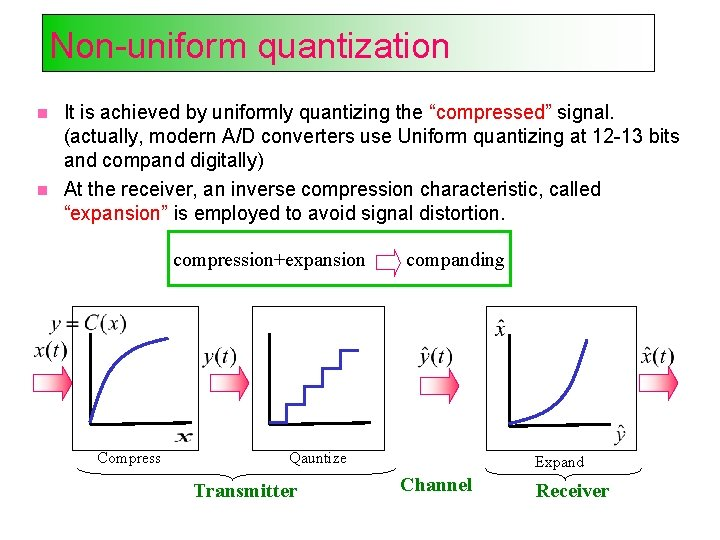Non-uniform quantization It is achieved by uniformly quantizing the “compressed” signal. (actually, modern A/D converters use Uniform quantizing at 12 -13 bits and compand digitally) At the receiver, an inverse compression characteristic, called “expansion” is employed to avoid signal distortion. compression+expansion Compress companding Qauntize Transmitter Expand Channel Receiver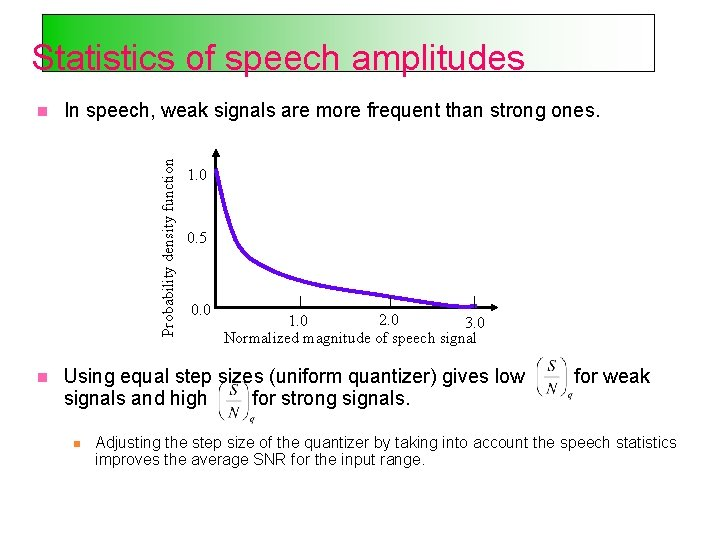Statistics of speech amplitudes In speech, weak signals are more frequent than strong ones. Probability density function 1. 0 0. 5 0. 0 2. 0 1. 0 3. 0 Normalized magnitude of speech signal Using equal step sizes (uniform quantizer) gives low signals and high for strong signals. for weak Adjusting the step size of the quantizer by taking into account the speech statistics improves the average SNR for the input range.Baseband transmission To transmit information through physical channels, PCM sequences (codewords) are transformed to pulses (waveforms). Each waveform carries a symbol from a set of size M. Each transmit symbol represents bits of the PCM words. PCM waveforms (line codes) are used for binary symbols (M=2). M-ary pulse modulation are used for non-binary symbols (M>2).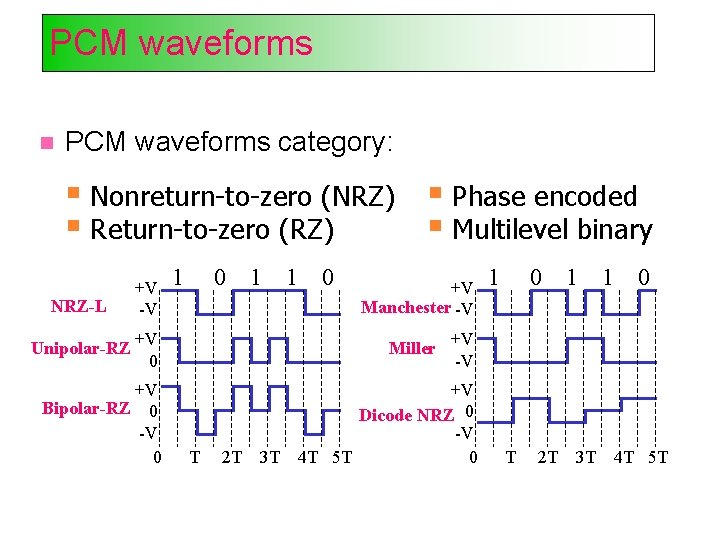PCM waveforms category: Nonreturn-to-zero (NRZ) Phase encoded Return-to-zero (RZ) Multilevel binary NRZ-L +V -V 1 0 1 1 0 +V Manchester -V Unipolar-RZ +V 0 Miller +V -V +V Bipolar-RZ 0 -V 0 +V Dicode NRZ 0 -V 4 T 5 T 0 T 2 T 3 T 1 0 T 2 T 1 3 T 1 0 4 T 5 TPCM waveforms … Criteria for comparing and selecting PCM waveforms: Spectral characteristics (power spectral density and bandwidth efficiency) Bit synchronization capability Error detection capability Interference and noise immunity Implementation cost and complexitySpectra of PCM waveformsM-ary pulse modulation M-ary pulse modulations category: M-ary pulse-amplitude modulation (PAM) M-ary pulse-position modulation (PPM) M-ary pulse-duration modulation (PDM) M-ary PAM is a multi-level signaling where each symbol takes one of the M allowable amplitude levels, each representing bits of PCM words. For a given data rate, M-ary PAM (M>2) requires less bandwidth than binary PCM. For a given average pulse power, binary PCM is easier to detect than M-ary PAM (M>2).PAM example